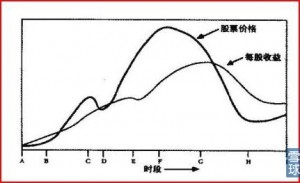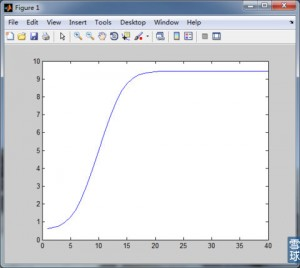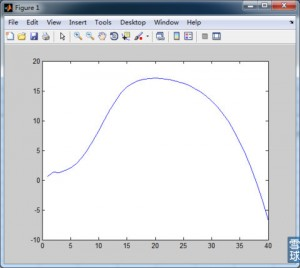# 招看穿一切旁氏骗局——泡沫以及复利的魔力《转自雪球》n 为参与的次数
O(n) 函数为出金的函数，而出金的数量和入金的比值，称为该月出金意愿，用函数P(n)来表示。

N(n)=I(n)-O(n)=I(n)-[P(n)*N(n-1)+N(n-1)*r]

syms x; f1=exp(-((x-10)/5)^2); s1=int(f1,x); s1=simple(s1)P=(x-10)^2/500;erO(1)=0;
I(1)=(5*pi^(1/2)*erf(1/5 – 2))/2+5;
N(1)=I(1); P(1)=(1-10)^2/500;
for i=2:40
MO=((5*pi^(1/2)*erf((i-1)/5 – 2))/2+5-O(1))*(0.1+(i-1-10)^2/500);
O(i)=MO;
I(i)=(5*pi^(1/2)*erf(i/5 – 2))/2+5;
P(i)=(i-10)^2/500;
N(i)=I(i)+(0.9+P(i-1))*(I(i-1)-O(i-1));
end（一）融资能力到达上限，借不到更多的钱维系。那么只要看参与者的融资杠杆率开始下降即可知道拐点会到来。

（二）杠杆率被强制降低，如果央行加息等因素导致融资的情况突然发生变化，则拐点将突然到来。